# LaTeX 插入高亮代码（LaTex、Python、Java、C、C++等主流语言都支持）

## 我为什么要介绍两个包？

\documentclass{article}
\usepackage{listings}
\begin{document}

\begin{lstlisting}[language = tex]
\begin{lstlisting}[language=Python]
import numpy as np
print(np.ones(5)
\end{lstlisting}
\end{lstlisting}
\end{document}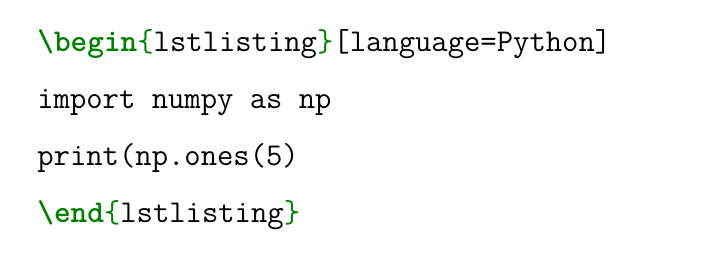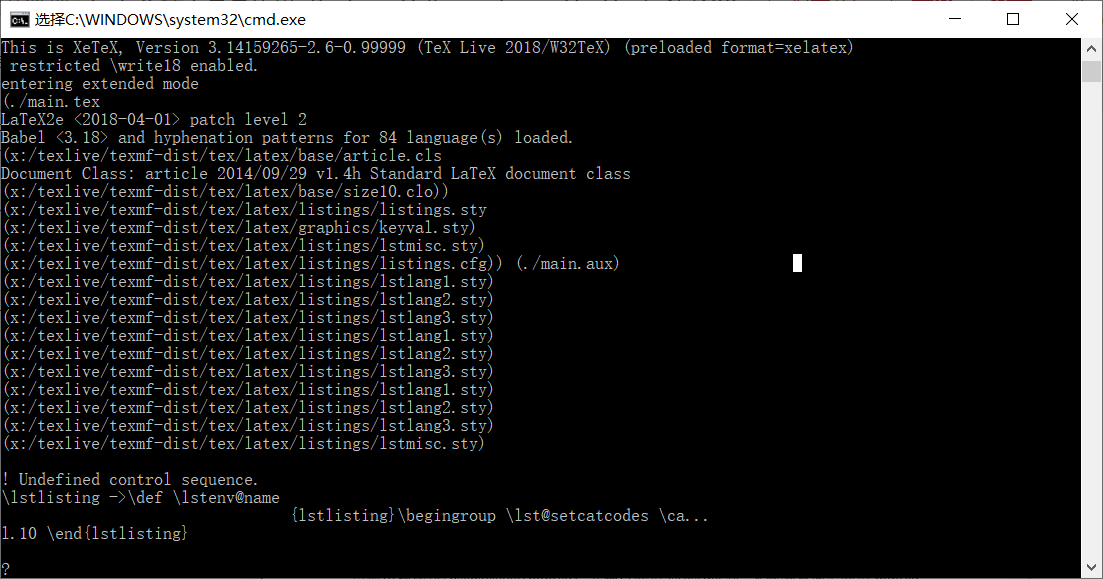\documentclass{article}
\usepackage{listings}
\usepackage{minted}
\usepackage{xcolor}
\begin{document}
code show：
\begin{minted}{tex}
\begin{lstlisting}[language=Python]
import numpy as np
print(np.ones(5)
\end{lstlisting}
\end{minted}
\end{document}


## minted

### 环境配置

minted包依赖于xcolor包，依赖python环境以pygents包，在编译命令时还需要加入-shell-escape参数。Python环境以及pygents包(pip install pygents即可)配置对于有一定python基础的盆友就很简单了，对于上一节中的main.tex文件，编译的命令:xelatex -shell-escape main，别忘了要导入宏包\usepackage{minted}。如果你使用的是texstudio的话，配置起来也简单如图：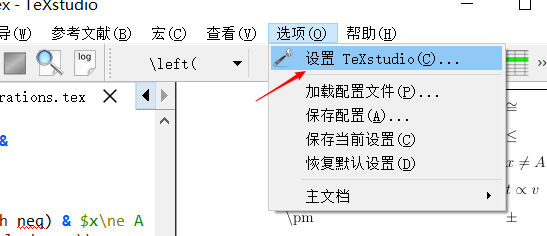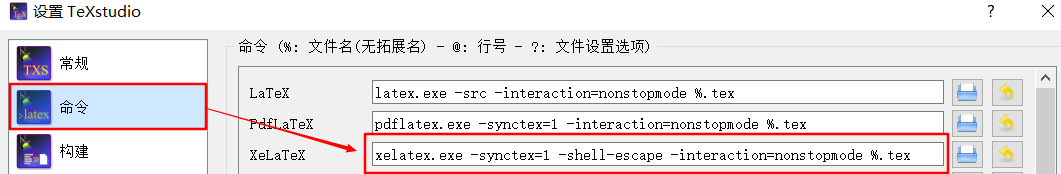### 基本使用

\begin{minted}[options]{<language>}
<code>
\end{minted}


\mint{python}|import numpy as np|


使用\mintinline{python}{import numpy as np}导入即可


\inputminted[<options>]{<language>}{<filename>}.


## lstlisting

\usepackage{listings}
\newfontfamily\courier{Courier New}
\lstset{linewidth=1.1\textwidth,
numbers=left, %设置行号位置
basicstyle=\small\courier,
numberstyle=\tiny\courier, %设置行号大小
keywordstyle=\color{blue}\courier, %设置关键字颜色
%identifierstyle=\bf，
stringstyle=\it\color[RGB]{128,0,0}\courier,
%framexleftmargin=10mm,
frame=single, %设置边框格式
backgroundcolor=\color[RGB]{245,245,244},
%escapeinside=, %逃逸字符(1左面的键)，用于显示中文
breaklines, %自动折行
extendedchars=false, %解决代码跨页时，章节标题，页眉等汉字不显示的问题
xleftmargin=2em,xrightmargin=2em, aboveskip=1em, %设置边距
tabsize=4, %设置tab空格数
showspaces=false %不显示空格
basicstyle=\small\courier
}


\begin{lstlisting}[language = tex]
\begin{minted}[frame=lines,
framesep=2mm,
baselinestretch=1.2,
fontsize=\footnotesize,
linenos]{python}
import numpy as np
print(np.ones(1))
\end{minted}
\end{lstlisting}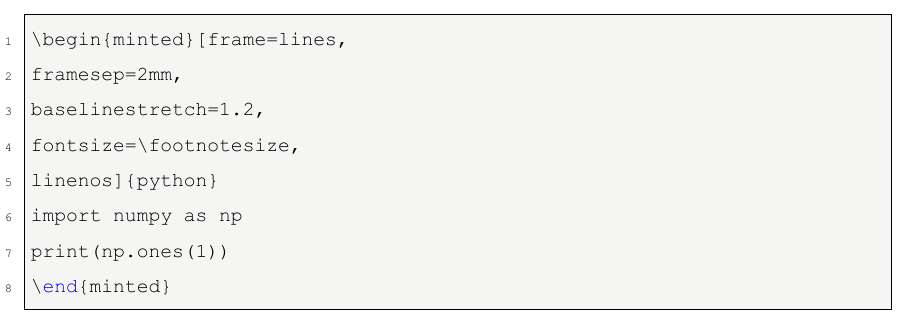## 总结

11-26
07-2752510-03227
11-10431
01-241万+
09-20300
10-091万+
09-306万+
10-266234
10-221万+